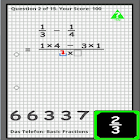Basic Fractions - Maths

All Android applications categories

All Android games categories# Basic Fractions - Maths

by: 0 0

## Screenshots

Description

Many different techniques can be adopted to solve fractions. Using some such techniques - and active user involvement - Basic Fractions will show the user how to add, subtract, multiply and divide normal and improper fractions.

The application is split into four categories (Addition, Subtraction, Multiplication and Division), and there are a total of 70 questions.

At the bottom of the screen for each question is a list a numbers from which the user 'drags and drops' the answers into the highlighted answer location. Once the correct/acceptable answer is 'dropped', the next answer location will become available. This continues until the question is completely answered.

Basic Fractions contains context-related help screens; offering help relevant to where the user is at that point.

Basic Fractions covers normal and improper fractions, but does not simplify the answers (e.g., into whole numbers and fractions).